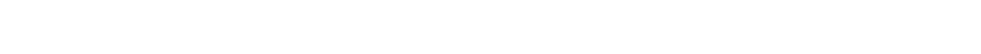#### Heat Energy Change

Heat energy change:

Data from a calorimetry experiment can be used to calculate the heat energy change of a reaction.

Equation:Q – energy transferred to water

m – mass of water heated *if water is not used, this is replaced by the mass or volume of other solutions

c –  the specific heat capacity – is the amount of heat needed to raise the temperature of 1 gram of a substance by 1 oC. For water, the value is 4.18 J g-1C-1 (joules per gram per degree Celsius).

∆T – change in temperature of water

Example:

The energy from burning 0.5 g of propane was transferred to 100 cm3 of water to raise its temperature by 20°C. Calculate the heat energy change (in kJ)

Mass of Water =     100 cm3

Heat capacity of Water =     4.2 j / g / C

Temperature rise     =     20°C

Energy transferred     =     100    x    4.2    x    20    =    8400 J

*1000 J = 1 kJ

So 8400 J   =  8.4 kJ

Energy transferred = 8.4 kJ

## Aiming for a Level 9?

See if you’ve got what it takes. Test yourself with our topic questions.

### Author: Jamie

Jamie got a First class degree in Chemistry from Oxford University before going on to teach chemistry full time as a professional tutor. He’s put together these handy revision notes to match the Edexcel IGCSE Chemistry specification so you can learn exactly what you need to know for your exams.